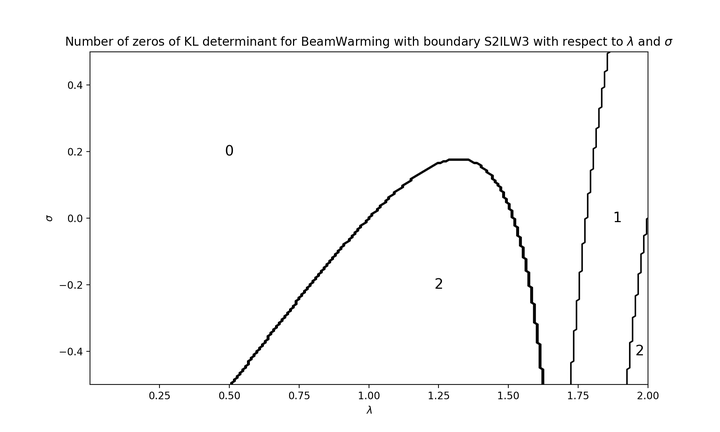# On the stability of totally upwind schemes for the hyperbolic initial boundary value problem### Résumé

In this paper, we present a numerical strategy to check the strong stability (or GKS-stability) of one-step explicit totally upwind schemes in 1D with numerical boundary conditions. The underlying approximated continuous problem is the one-dimensional advection equation. The strong stability is studied using the Kreiss-Lopatinskii theory. We introduce a new tool, the intrinsic Kreiss-Lopatinskii determinant, which possesses remarkable regularity properties. By applying standard results of complex analysis, we are able to relate the strong stability of numerical schemes to the computation of a winding number, which is robust and cheap. The study is illustrated with the Beam-Warming scheme together with the simplified inverse Lax-Wendroff procedure at the boundary.

Type
Publication
IMA Journal of Numerical Analysis (in press)